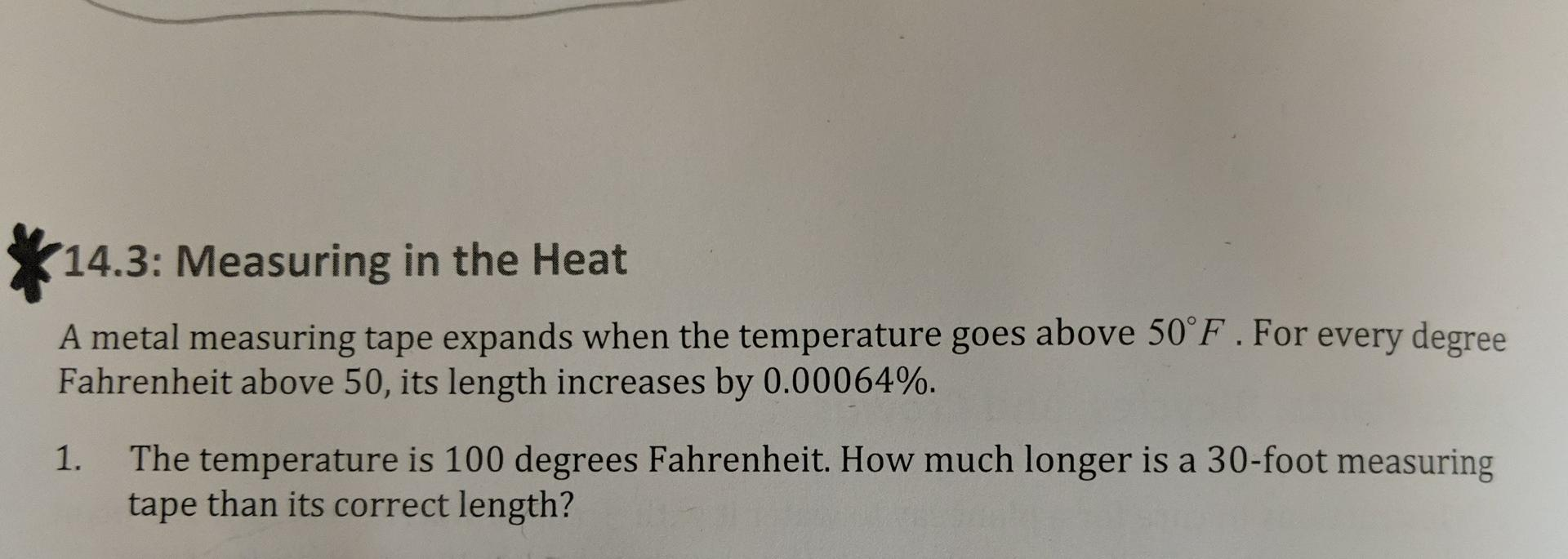# (Solved):   14.3: Measuring in the Heat A metal measuring tape expands when the temperature goes above ...14.3: Measuring in the Heat A metal measuring tape expands when the temperature goes above $$50^{\circ} \mathrm{F}$$. For every degree Fahrenheit above 50, its length increases by $$0.00064 \%$$. 1. The temperature is 100 degrees Fahrenheit. How much longer is a 30 -foot measuring tape than its correct length?

We have an Answer from Expert